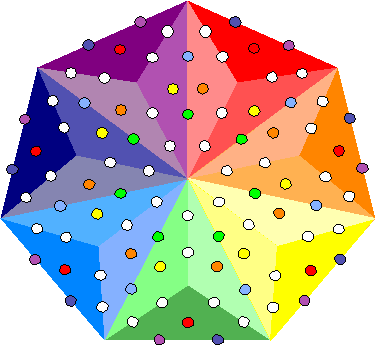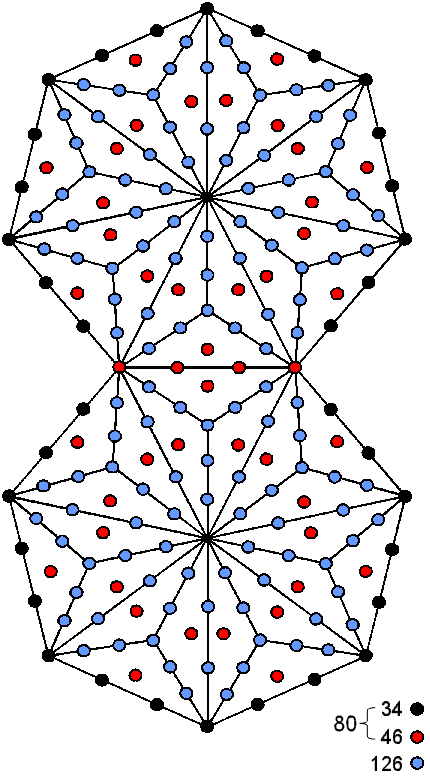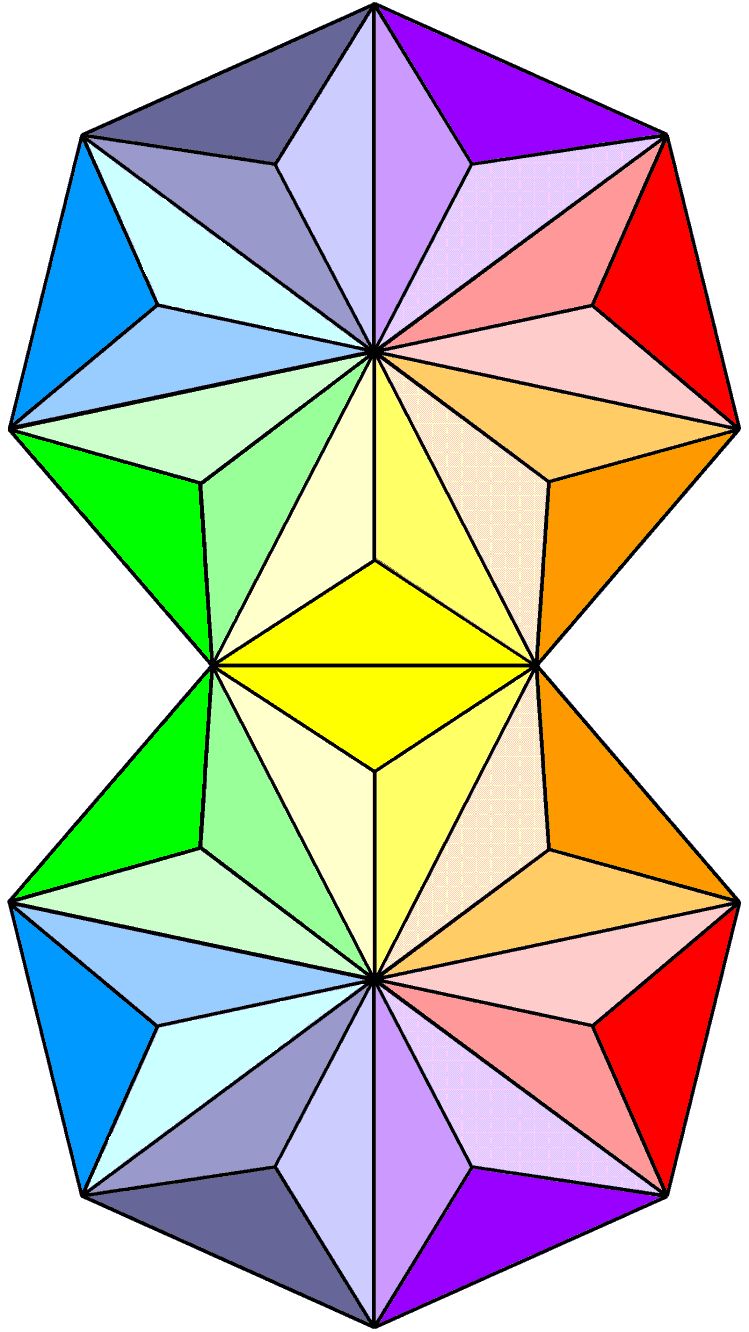Home

 << Previous    1...   8  9    11  12  ...15    Next >>

#10 The 206 yods surrounding the centres of two joined Type B heptagons symbolize the 206 bones of the human skeleton

The triangle and square have seven corners. The spiritual cosmos is sevenfold in that it comprises seven cosmic planes of consciousness (see Maps of reality/Cosmic Tetractys). The seven-cornered heptagon is absent from the set of seven regular polygons that make up half of the inner form of the Tree of Life. Yet it encodes the number (91) of Trees of Life in the Cosmic Tree of Life as the 91 hexagonal yods contained in its seven sectors when they are Type A triangles (Fig. 14). The 49 coloured hexagonal yods symbolize the 49 Trees of Life that map the 49 subplanes of the cosmic physical plane and the 42 white hexagonal yods symbolize the 42 subplanes of the six cosmic superphysical planes.Figure 15. The 206 yods surrounding the centres of two joined, Type B heptagons symbolize the 206 bones in the human skeleton. The 34 black yods on their boundary symbolize the 34 single bones in the axial skeleton. The two sets of 23 red yods symbolize its 23 pairs of bones. The two sets of 63 blue yods denote the 63 pairs of bones in the appendicular skeleton. The 80 black & red yods in the two joined, Type A heptagons symbolise the80 bones in the human axial skeleton; the additional 126 blue yods in the two joined, Type B heptagons symbolise the 126 bones in the human appendicular skeleton. Figure 14. The Type B heptagon has 91 hexagonal yods.

85 yods line the 35 sides of the 21 triangles in a Type B heptagon, where

85 = 40 + 41 + 42 + 43.

(40=1) denotes its centre, which is surrounded by 84 such boundary yods, where

84 = 12 + 32 + 52 + 72.

Two separate Type B heptagons have 210 (=21×10) yods surrounding their centres, of which 168 yods line the 70 sides of their 42 triangles. This is how the heptagon embodies the superstring structural parameter 168. Two joined, Type B heptagons (Figure 15) have 206 yods surrounding their centres. They comprise:

• 34 black yods lining their unjoined sides and symbolizing the 34 single bones in the human axial skeleton;
• 23 pairs of red yods that symbolize the 23 pairs of bones in the human axial skeleton;
• two sets of 63 blue yods (i.e., 63 pairs) that symbolize the 63 pairs of bones in the human appendicular skeleton.

(see also #9 here). Just as 21 regular polygons with 103 associated corners are enfolded on each side of the central Pillar of Equilibrium of the 3-tree as its inner form, so 21 tetractyses with 103 associated yods surround the centre of each heptagon. The heptagon is the measure of not only the spiritual cosmos but also the human skeleton, the two joined, Type A versions expressing the 80 bones in the axial skeleton and the 126 extra yods in the two joined, Type B heptagons denoting the 126 bones of the appendicular skeleton. The number 103 is the number value of SABAOTH ("Hosts"), part of the Godnames of Hod and Netzach (see Table 1 in #2).

As the early Theosophists taught, e.g., see Annie Besant's The Seven Principles of Man (download PDF here), a human being embodies seven principles. Amazingly, the bones in the human skeleton can be represented by two joined, Type B heptagons, that is, two seven-sided polygons. When they are Type A, their 80 black & red yods symbolize the 80 bones of the human axial skeleton; when they are Type B, their additional 126 blue yods denote the 126 bones in the human appendicular skeleton. Remarkably, the Type A/Type B distinction between polygons expresses the difference between the two types of human skeletons when the polygon is a heptagon.Figure 16. 137 corners, sides & triangles surround the centres of two joined, Type B heptagons embodying 206 yods.

The number of corners, lines & triangles in a Type B n-gon = 10n +1, where "1" denotes its centre (see here). A Type B heptagon (n=7) comprises 70 geometrical elements surrounding its centre. 67 geometrical elements outside one side with two corners & one side, i.e., three geometrical elements, surround its centre. 67 is the number of Binah, the third Sephirah. Two joined, Type B heptagons have (3 + 2×67 = 137) geometrical elements surrounding their centres (Fig. 16). Their 206 yods belong to (21+21=42) triangles having 26 corners and 69 sides surrounding the centres of the heptagons, i.e., 95 corners & sides. 26 is the number of YAHWEH and 95 is the number of Madim, the Mundane Chakra of Geburah. This chance-defying conjunction of the number of bones in the human skeleton and the number 137 determining the fine-structure constant (one of the most mysterious numbers in atomic, nuclear & particle physics) is amazing.

We conclude that the heptagon embodies not only the superstring structural parameters 84 & 168 but also the fine-structure number 137 and the number of bones in the human skeleton.

 << Previous    1...   8  9    11  12  ...15    Next >>

Home